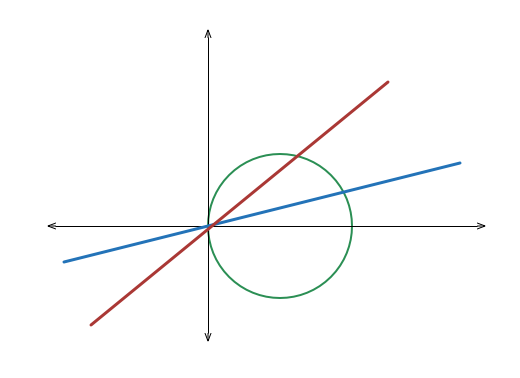# Any way you slice it

Geometry Level 5Suppose that two distinct lines passing through the origin cut through the region $R$ in the first quadrant bounded by the $x$-axis and the circle $(x - 1)^{2} + y^{2} = 1$ such that the lines divide $R$ into $3$ subregions of equal area.

If the sum of the slopes of these two lines is $S$, find $\lceil 1000*S \rceil$.

Note: Numerical methods can be used in the process of solving this question.

×